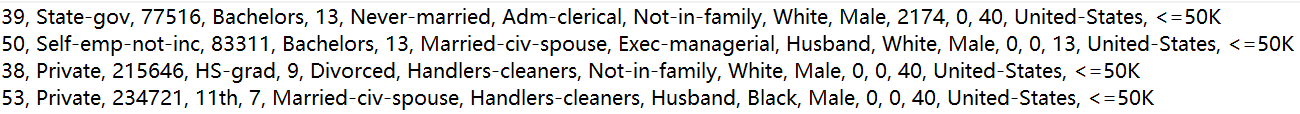# 数据信息

（下载的数据中没有标题，标题可有可无）

'age', 'workclass', 'fnlwgt', 'education', 'education_num',
'marital_status', 'occupation', 'relationship', 'race', 'gender',
'capital_gain', 'capital_loss', 'hours_per_week', 'native_country',
'income_bracket'# 流程

sklearn.preprocessing.LabelEncoder 类可以在不损失数据的情况下对数据进行编码和解码

• 数据加载与处理
• 创建模型
• 训练模型
• 评判模型
• 使用模型预测新数据

# 开始编码

## 导入需要用到的类和方法

# 用于编码的类
from sklearn.preprocessing import LabelEncoder

# 朴素贝叶斯类
from sklearn.naive_bayes import GaussianNB

# 交叉验证方法
from sklearn.model_selection import cross_val_score

import numpy as np


## 数据加载与处理

less_than_50k = []
more_than_50k = []

# 记录一下含有空缺值的数据
blank_value = 0

# 若某行数据有缺失值，则舍弃掉这条数据
if ' ?' in line:
blank_value += 1
continue
# data 是一行的数据，每一行的最后一个是 \n 换行符，所以是 line[:-1]，表示不处理换行符
# 注意这里分隔符是 英文逗号加一个空格，建议直接在文件中复制
data = line[:-1].split(', ')
if data[-1] == '<=50K':
less_than_50k.append(data)
# 注意这里不能使用 else ，因为文件末尾有几行没有数据
elif data[-1] == '>50K':
more_than_50k.append(data)


less_than_50k_length = len(less_than_50k)
more_than_50k_length = len(more_than_50k)

print(less_than_50k_length)  # 22654
print(more_than_50k_length)  # 7508
print(blank_value)  # 2399


# 比较大于50k和不大于50k的数据量，从两个类别的数据中提取出相同数据量
if less_than_50k_length == more_than_50k_length:
pass
elif less_than_50k_length > more_than_50k_length:
less_than_50k = less_than_50k[:more_than_50k_length]
else:
more_than_50k = more_than_50k[:less_than_50k_length]


# 先将两个list转换为 numpy的二维数组，再使用np.vstack将两个二维数组合并为一个大的二维数组
less_ndarray = np.array(less_than_50k)
more_ndarray = np.array(more_than_50k)


# 将两个二维数组合并为一个大的二维数组
data = np.vstack([less_ndarray, more_ndarray])


# 查看合并和的二维数组的形状，检验是否合并成功
print(data.shape)
# (15016, 15)


## 对样本特征值进行编码

# 标签编码器列表
label_encoder = []

# 存放编码后的数据，先指定为空的二维数组，再进行赋值
data_encoder = np.empty(data.shape)

# index是data每一个元素的下标，item是data每一个元素的内容
for index, item in enumerate(data):
# 若该列数据是是数字，直接赋值，否则先进行编码
if item.isdigit():
data_encoder[:, index] = data[:, index]
else:
# 对于每一个非数字的列，分别创建一个标签编码器，便于后期用来预测样本
# 每一个标签编码器分别使用各自列的数据进行编码，这样预测数据时可以不用再训练标签分类器，直接对需要预测的样本数据进行编码
label_encoder.append(LabelEncoder())
data_encoder[:, index] = label_encoder[-1].fit_transform(data[:, index])


X_train = data_encoder[:, :-1].astype(int)
y_train = data_encoder[:, -1].astype(int)


## 模型的创建与训练

# 创建朴素贝叶斯分类器
gnb_clf = GaussianNB()
# 使用样本数据训练朴素贝叶斯模型
gnb_clf.fit(X_train, y_train)


## 评判模型

cv = 10 表示交叉验证将数据分为10组

# 使用交叉验证对模型进行评判
scores = cross_val_score(gnb_clf, X_train, y_train, cv=10)

print(f"交叉验证得分：{round(np.mean(scores), 3)}")
# 交叉验证得分：0.627


## 使用模型预测新数据

### 对新数据特征值进行编码

# 创建一个新样本，使用训练好的模型对其进行预测，预测该样本的收入类别
input_data = ['39', 'State-gov', '77516', 'Bachelors', '13', 'Never-married', 'Adm-clerical', 'Not-in-family', 'White',
'Male', '2174', '0', '40', 'United-States']

# 先初始化一个列表，用于存放新样本数据编码后的结果
input_data_encoder = [-1] * len(input_data)

# 对新样本的数据进行编码

# count表示新样本数据中非数字的列的顺序
# 在上面我们有一个训练好的编码器列表，通过count使用非数值的列对应的编码器对新样本数据进行编码
count = 0
for index, item in enumerate(input_data):
if item.isdigit():
input_data_encoder[index] = int(item)
else:
# tranform()对数据进行编码，参数是一个 numpy 的 ndarray，所以需要使用 np.array() 构建一个ndarray
temp = label_encoder[count].transform(np.array([item]))
input_data_encoder[index] = int(temp)
count += 1

# 查看一下编码后的样本数据
print(input_data_encoder)
# [39, 5, 77516, 9, 13, 4, 0, 1, 4, 1, 2174, 0, 40, 37]

print(type(input_data_encoder))
# <class 'list'>


### 使用编码后的特征值进行预测

predict 的参数是一个 numpy 的二维数组，所以需要使用 reshape() 将其转换为二维数组

y_predict = gnb_clf.predict(np.array([input_data_encoder]).reshape(1,-1))


reshape(1,-1) 表示只有一个样本
reshape(-1,1) 表示样本只有一个特征值

y_predict 是经过编码后的数据，需要使用编码器解码
inverse_transform() 将编码后的数据进行解码，返回的是一个一维数组

print(label_encoder[-1].inverse_transform(y_predict))

# <=50K


# 编码器LabelEncoder小结

from sklearn.preprocessing import LabelEncoder


label = LabelEncoder()


label.fit_transform(X_data)


label.fit(X_data)


label.transform(X_data)


inverse_transform(X_encoder)


label.fit_transform(X_data) = label.fit(X_data) + label.transform(X_data)

07-09481
12-10133901-163563
12-032658
12-162138
12-24533
07-28
03-04
08-034180
01-171万+